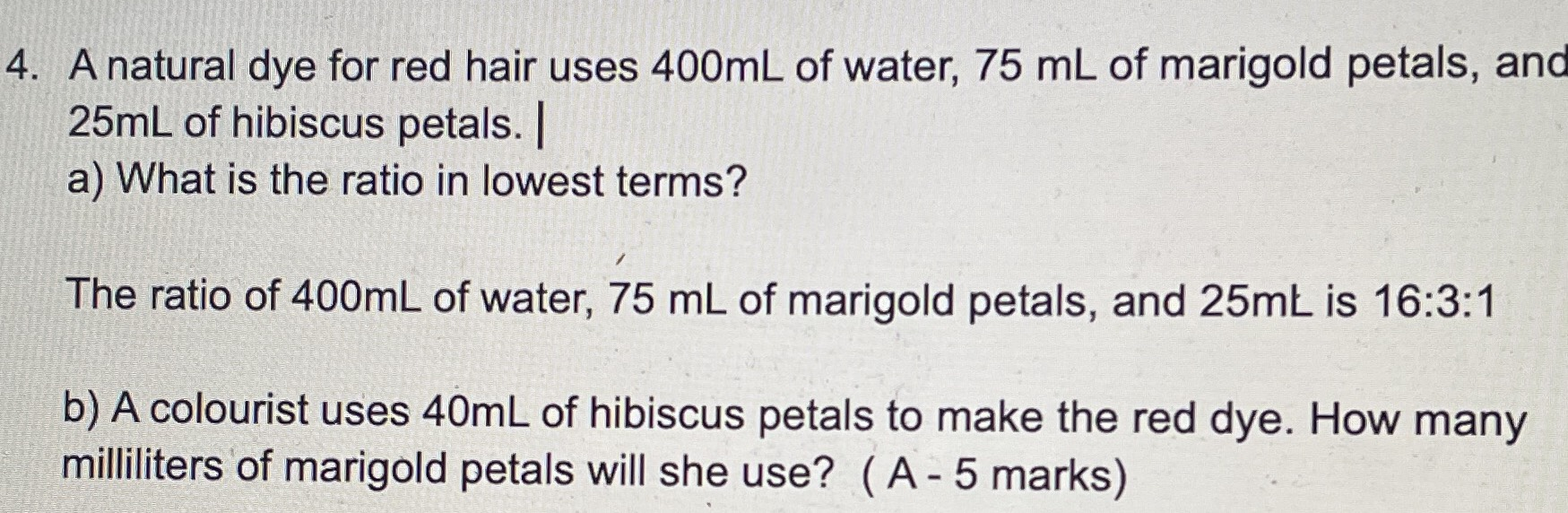### Still have math questions?

Arithmetic
QuestionA natural dye for red hair uses $$400 mL$$ of water, $$75 mL$$ of marigold petals, and $$25 mL$$ of hibiscus petals. a) What is the ratio in lowest terms? The ratio of $$400 mL$$ of water, $$75 mL$$ of marigold petals, and $$25 mL$$ is $$16 : 3 : 1$$

b) A colourist uses $$40 mL$$ of hibiscus petals to make the red dye. How many milliliters of marigold petals will she use? (A-5 marks)